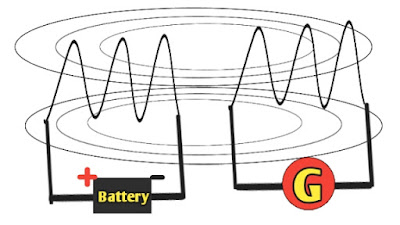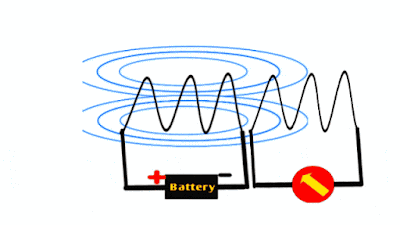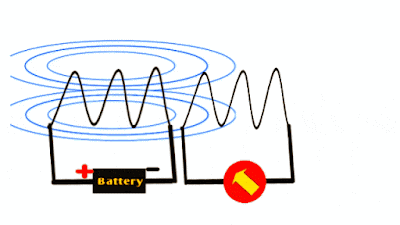# faraday's law of electromagnetic induction | electromagnetic induction

Hello guys, how are you? Hope you are all good and well.

• Definition for electromagnetic induction
• What is the magnetic field?
• What is magnetic flux?
• Faraday's law of electromagnetic induction.
• What is lenz law
• Applications of Faraday law of electromagnetic induction.

Now let's go to understand,

## Definition for electromagnetic induction: -

It states that whenever a magnetic field link with circuit changes, then an emf will induce in the circuit and if the circuit is close then a current will also flow into the circuit.

The emf is produced is known as induced emf.

So a question arises here,

### What is a magnetic field?

The attractive or repulsive force around a magnet is known as the magnetic field.

### What is magnetic flux: -

The total quantity of magnetic field produced by a magnet around it is known as magnetic flux. It is measured in Weber (wb).

Now let's go to understand,

## Faraday experiment on electromagnetic induction: -

Let understand this into three cases.

Experiment apparatuses: -

• One coil to which a galvanometer is connected.
• Another coil to which a battery (DC supply) is connected.

Case 1: -

When Coil 1 and 2 both are kept stationary.
In this case, coil 1 is connected to galvanometer and coil 2 is connected to the battery. The coil 2 will act as an electromagenet and produce magnetic fields of lines around it. This magnetic field will link with coil 1 but as both these coils are kept stationary, therefore there will no emf induce in the coil 1 either magnetic field (constant because DC is used) links with the coil.Fig. When both coil and electromagnet kept stationary

Case 2: -

Coil 1 kept stationary and coil 2 moving.
In this case, as discussed above the coil 2 is connected to DC source due to which it will produce a constant magnetic field around it.
As coil 2 is moving so its magnetic field will cut the coil 1. when coil 2 move towards to coil 1 an emf will induce in one direction, whereas when coil 2 moves away from the coil 1 it will an emf will again induce but in the opposite direction.Fig. Fig. When electromagnet is moving

Case 3: -

Coil 1 is moving and coil 2 is kept stationary.
This is the similar case as case 2 when it moves towards the electromagnet then emf induce in one direction and when it moves away from the electromagnet then it will induce an emf in another direction.Fig. When coil is moving

So in this experiment we can use both AC as well as DC. But the difference is that when we apply a DC supply and emf induced is called dynamic emf whereas when we apply AC supply an emf will induce and called static emf.Fig. faraday's law of electromagnetic induction with AC

In other words, we can say,

The emf induce by the relative motion between the coil and the electromagnet is known as dynamic induced emf.

This type of emf is induced in AC motor, generator (AC and DC both).

The emf induced without any relative motion between coil and the electromagnet is known as static induced emf.

This type of emf is induced in transformer.

From this experiment Faraday's conclude two laws, known as,

## 1. Faraday's first law: -

It states that when a magnetic field cuts a conductor (or coil) an emf will induce in the conductor.
Let assume a coil with galvanometer as shown below.Fig. faraday first law of electromagnetic induction

When the magnet moves towards the coil an emf will induce in one direction and when magnet moves away from the coil an emf will induce in the opposite direction as already discussed above.

This law tells us about the magnitude of an induced emf.

This law states that the magnitude of induced emf is directly proportional to the rate of change of flux link with the circuit.

If the rate of change is high means high emf will induce in the circuit.

Now let's go to understand the mathematical expression for the induced emf: -

Let assume the coil have N number of turns and rate of change of flux is Φ.

Then induced emf will given by,

The minus sign shows that the direction of induced emf is in opposition to the cause that produced it. (According to Lenz law)

Now a question arises here that,

## What is the lenz law?

Lenz law: -
This law is used to determine the direction of the induced emf in the coil when magnetic field cut across it.

This law states that the induced emf will oppose the cause that produced it.

For example,
When three phase AC supply is given to the induction motor then a current will flow through the stator.

Due to this current, a varying magnetic field will produce in the stator winding which will link with the rotor windings and induce an emf in the rotor.

The direction of this emf is in opposition to the stator current because it is produced due to the magnetic field of the stator which is further produced by current flowing in the stator.

## Applications of Faradays laws of electromagnetic induction: -

Now a day this law is almost used in all electrical machines like transformer, generator, motors etc.

In transformer a static emf is induced with the help of inuduction whereas in motor or generator a dynamic emf is induced.

It is also used in microphone and telephones.
Used in payment cards.

## Conclusion: -

To induce an emf in a coil or circuit, we require a varying magnetic field. A static magnetic field cannot produce any emf in the coil until a rotation or movement is not given to it.

When a coil links with varying magnetic field a emf will induce in the circuit and if the circuit is close then a current will also flow through it.

Faraday finds two most useful laws from his experiment (in 1831). These laws are known as faraday's law of electromagnetic induction.

If you have any doubt then tell me in a comment.

If you like the article then share with your friends.

Thank you.

Hope article helps you.